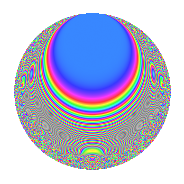# Properties

 Label 36.5.fLevel 36 Weight 5 Character orbit f Rep. character $$\chi_{36}(7,\cdot)$$ Character field $$\Q(\zeta_{6})$$ Dimension 44 Newform subspaces 1 Sturm bound 30 Trace bound 0

# Related objects

## Defining parameters

 Level: $$N$$ $$=$$ $$36 = 2^{2} \cdot 3^{2}$$ Weight: $$k$$ $$=$$ $$5$$ Character orbit: $$[\chi]$$ $$=$$ 36.f (of order $$6$$ and degree $$2$$) Character conductor: $$\operatorname{cond}(\chi)$$ $$=$$ $$36$$ Character field: $$\Q(\zeta_{6})$$ Newform subspaces: $$1$$ Sturm bound: $$30$$ Trace bound: $$0$$

## Dimensions

The following table gives the dimensions of various subspaces of $$M_{5}(36, [\chi])$$.

Total New Old
Modular forms 52 52 0
Cusp forms 44 44 0
Eisenstein series 8 8 0

## Trace form

 $$44q - q^{2} - q^{4} - 2q^{5} + 15q^{6} + 122q^{8} - 60q^{9} + O(q^{10})$$ $$44q - q^{2} - q^{4} - 2q^{5} + 15q^{6} + 122q^{8} - 60q^{9} + 28q^{10} - 228q^{12} - 2q^{13} + 252q^{14} - q^{16} - 56q^{17} + 72q^{18} - 140q^{20} + 138q^{21} - 33q^{22} - 951q^{24} - 1752q^{25} + 1096q^{26} - 516q^{28} + 526q^{29} - 1980q^{30} - 121q^{32} + 2994q^{33} + 385q^{34} - 1005q^{36} - 8q^{37} + 1395q^{38} - 2276q^{40} - 2762q^{41} + 3330q^{42} + 6714q^{44} + 4110q^{45} + 3576q^{46} + 2163q^{48} + 3428q^{49} + 6375q^{50} + 1438q^{52} - 10088q^{53} - 4983q^{54} - 7506q^{56} + 1752q^{57} - 4064q^{58} - 16392q^{60} - 2q^{61} - 18324q^{62} + 9026q^{64} - 2014q^{65} - 17358q^{66} - 11405q^{68} + 3354q^{69} + 3666q^{70} + 4083q^{72} - 3416q^{73} + 14620q^{74} + 1581q^{76} - 3942q^{77} + 34566q^{78} + 45520q^{80} - 1164q^{81} - 8486q^{82} + 51078q^{84} - 1252q^{85} + 22113q^{86} + 1995q^{88} + 13048q^{89} - 4692q^{90} - 30294q^{92} + 12090q^{93} + 7524q^{94} - 76164q^{96} + 5638q^{97} - 92938q^{98} + O(q^{100})$$

## Decomposition of $$S_{5}^{\mathrm{new}}(36, [\chi])$$ into newform subspaces

Label Dim. $$A$$ Field CM Traces $q$-expansion
$$a_2$$ $$a_3$$ $$a_5$$ $$a_7$$
36.5.f.a $$44$$ $$3.721$$ None $$-1$$ $$0$$ $$-2$$ $$0$$

## Hecke characteristic polynomials

There are no characteristic polynomials of Hecke operators in the database